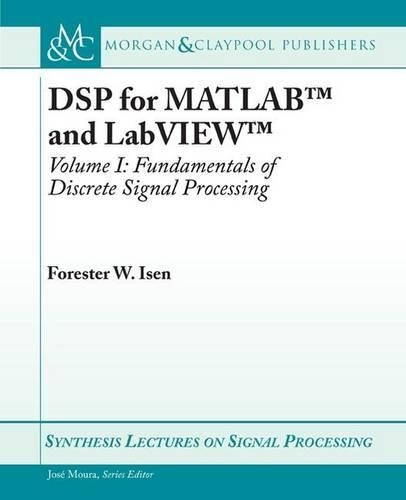•DSP for MATLAB ™ and LabVIEW ™ I: Fundamentals of Discrete Signal Processing - Synthesis Lectures on Signal Processing (Paperback)

(author)
£41.95
Paperback 213 Pages / Published: 30/01/2009
• We can order this

Usually dispatched within 3 weeks

This book is Volume I of the series DSP for MATLAB ™ and LabVIEW ™. The entire series consists of four volumes that collectively cover basic digital signal processing in a practical and accessible manner, but which nonetheless include all essential foundation mathematics. As the series title implies, the scripts (of which there are more than 200) described in the text and supplied in code form here will run on both MATLAB and LabVIEW. Volume I consists of four chapters. The first chapter gives a brief overview of the field of digital signal processing. This is followed by a chapter detailing many useful signals and concepts, including convolution, recursion, difference equations, LTI systems, etc. The third chapter covers conversion from the continuous to discrete domain and back (i.e., analog-to-digital and digital-to-analog conversion), aliasing, the Nyquist rate, normalized frequency, conversion from one sample rate to another, waveform generation at various sample rates from stored wave data, and Mu-law compression. The fourth and final chapter of the present volume introduces the reader to many important principles of signal processing, including correlation, the correlation sequence, the Real DFT, correlation by convolution, matched filtering, simple FIR filters, and simple IIR filters. Chapter 4, in particular, provides an intuitive or ""first principle"" understanding of how digital filtering and frequency transforms work, preparing the reader for Volumes II and III, which provide, respectively, detailed coverage of discrete frequency transforms (including the Discrete Time Fourier Transform, the Discrete Fourier Transform, and the z-Transform) and digital filter design (FIR design using Windowing, Frequency Sampling, and Optimum Equiripple techniques, and Classical IIR design). Volume IV, the culmination of the series, is an introductory treatment of LMS Adaptive Filtering and applications. The text for all volumes contains many examples, and many useful computational scripts, augmented by demonstration scripts and LabVIEW Virtual Instruments (VIs) that can be run to illustrate various signal processing concepts graphically on the user's computer screen.

Publisher: Morgan & Claypool Publishers
ISBN: 9781598298901
Number of pages: 213
Weight: 414 g
Dimensions: 235 x 187 x 12 mm